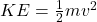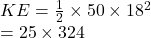## What is the kinetic energy of an object that has a mass of 50.0 kg and a velocity of 18 m/s? 450 J 900 J 8,100 J

Question

What is the kinetic energy of an object that has a mass of 50.0 kg and a velocity of 18 m/s?

450 J
900 J
8,100 J
16,000 J

in progress 0
2 months 2021-07-15T07:36:52+00:00 2 Answers 2 views 0

## The answer is 8,100 J

Explanation:

The kinetic energy of a body when given it’s mass and velocity can be found by using the formulawhere

m is the mass

v is the velocity

From the question

mass of object = 50 kg

velocity = 18 m/s

It’s kinetic energy isWe have the final answer as

### 8100 J

Hope this helps you